# Java Double and NaN WeirdnessBy Dirk van Rensburg | Senior Consultant

We learn something every day. We don’t always realise it, but we do. Sometimes the thing you learn isn’t new at all, but something you knew a little about, but never really thought about too much.

I recently learned that my understanding of what causes a NaN value in Java’s double was wrong.

## The story

I was working on an integration project and received a bug report on one of my services. The report said that my service is returning an HTTP code ‘500’ for a specific input message.

During my investigation, I found the cause of the exception was an unexpected value returned from a down stream service. It was a SOAP service which returned something like the following in its XML response:I was a bit surprised to see the NaN there since I would expect them to either leave the field off or set it to null if they don’t have a value. This looked like a calculation bug since we all know that, in Java and C# at least, dividing a double with 0 results in a NaN. (Spoiler: It doesn’t)

However, this got me thinking and I tried to remember what I know about double and NaN. This resulted in an embarrassingly deep spiral down the rabbit hole.

## NaN

Well if you think about it NaN is kind of like a number in this case, even though NaN means Not-a-Number. It exists to enable calculations with indeterminate results to be represented as a “number” in the set of valid double values. Without NaN you could get completely wrong results or you’ll get an exception, which isn’t ideal either. NaN is defined, same as Infinity, to be part of the set of valid doubles.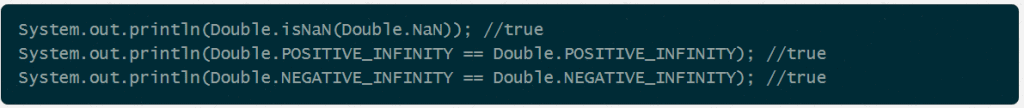I played around with double a bit and I thought to share it in a post, because I think the various edge cases of double are interesting.

I started with the following experiment: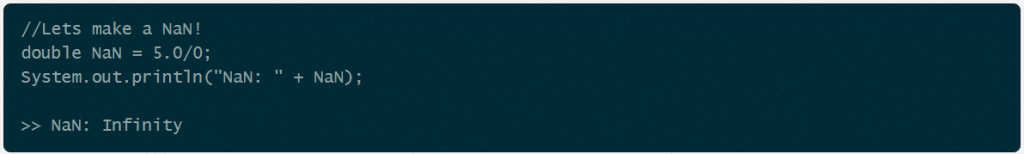## Wait. What?

Turns out that I have lived with this misconception about what happens when you divide a double by zero. I seriously expected that a double divided by 0 is NaN. Well it turns out I was wrong. You get:

POSITIVE_INFINITY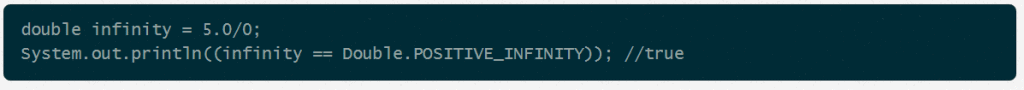I can sort of rationalise that the answer could be infinity because you are dividing something largish with something much much smaller. In fact, dividing it by nothing so you could argue the result of that should be infinitely large. Although, mathematically this does not make any sense. x/0 is undefined since there is no number that you can multiply with 0 to get back to x again. (for x <> 0)

Anyway let’s play with NaN a bit.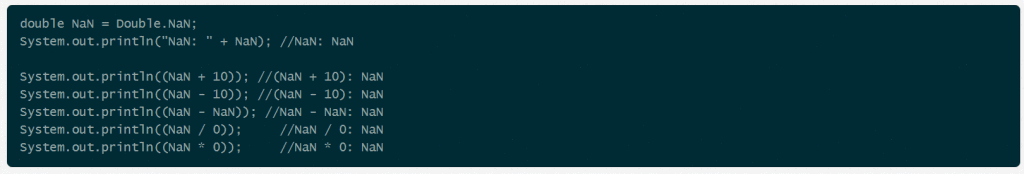Well no surprises here. Once a NaN always a NaN.

I used Double.NaN above to be sure I have a NaN but if you want to make one yourself then calculating the square root of a negative number is an easy way: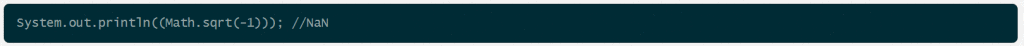## Max and Min value

Before we get to infinity let take a quick look at Double.MAX_VALUE and Double.MIN_VALUE. These are special constants defined on Double which you can use to check if a number is at the maximum of what a double can represent. If a number is equal to Double.MAX_VALUE it means that it is about to overflow into Double.POSITIVE_INFINITY. The same goes for Double.MIN_VALUE except that it will overflow to Double.NEGATIVE_INFINITY.

Something to note about double is that it can represent ridiculously large numbers using a measly 64 bits. The maximum value is larger than 1.7*10^308 !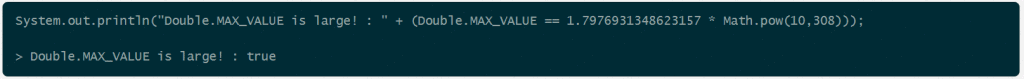It can represent these large numbers because it encodes numbers as a small real number multiplied by some exponent. See the IEEE spec

Let’s see what it takes to make Double.MAX_VALUE overflow to infinity.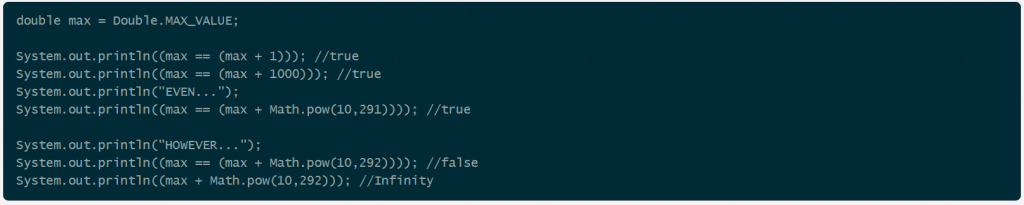This ability to represent seriously large numbers comes at a price of accuracy. After a while only changes in the most significant parts of the number can be reflected. As seen in the following code snippet: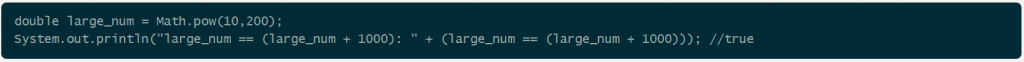At large integer values the steps between numbers are very very large since the double has no place to record the change if it doesn’t affect its most 16 most significant digits. As shown above 1000 plus a very large number is still that same very large number.

## Infinity

Java’s double supports two kinds of infinity. Positive and negative infinity. The easiest to make those are by dividing by 0.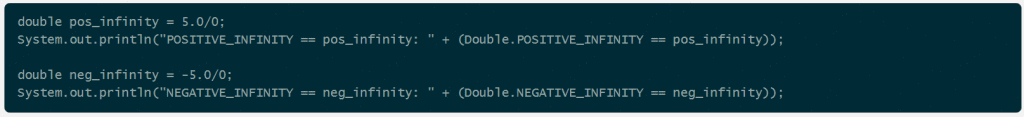In maths infinity is a numerical concept representing the idea of an infinitely large number. It is used, for example in calculus, to describe an unbounded limit – some number that can grow without bound.

In this case things are pretty much the same as in maths, where POSITIVE_INFINITY and NEGATIVE_INFINITY are used to represent numbers that are infinitely large. However, they function more as a way to know something went wrong in your calculation. You are either trying to calculate something that is too large to store in a double or there is some bug in the code.

There are once again some interesting things to note when playing with positive and negative infinity.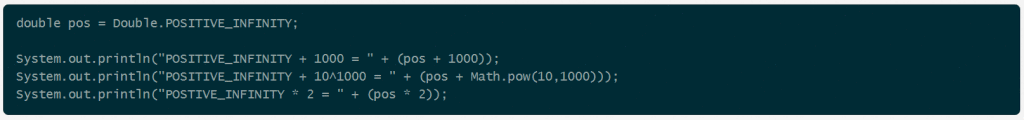Once the value is infinity it stays there even if you add or subtract ridiculously large numbers. However, there is one interesting case, when you subtract infinity from infinity: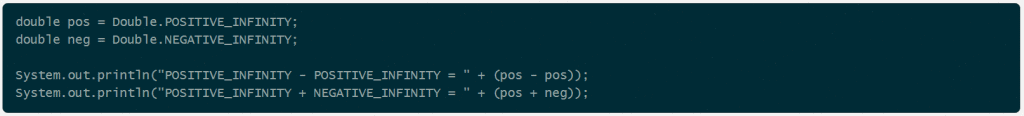Subtracting infinity from infinity yields NaN and as you would expect adding or subtracting NaN yields a NaN again.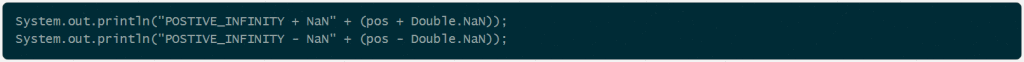## In closing

Both Java’s float and double types follow the IEEE 754-1985 standard for representing floating point numbers. I am not going to go into great detail on the internals of double, but it suffices to say that double and float are not perfectly accurate when you use them to perform arithmetic. The Java primitive type documentation says:

This data type should never be used for precise values, such as currency. For that, you will need to use the java.math.BigDecimal class instead.

If precision is you main concern then it is generally better to stick with good old java.math.BigDecimal. BigDecimal is immutable which makes it nice to work with, but the most important thing is precision. You have absolute control over number precision, without the rounding or overflow surprises you get with double and float. However, if performance is the main concern it is better to stick with float or double and live with the inaccuracies.